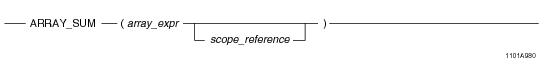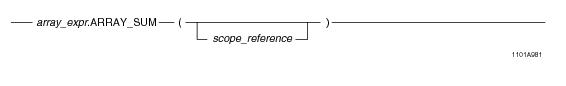# 15.00 - ARRAY_SUM - Teradata Database

## Teradata Database SQL Functions, Operators, Expressions, and Predicates

Product
Release Number
15.00
Content Type
Programming Reference
Publication ID
B035-1145-015K
Language
English (United States)

## ARRAY_SUM

### Purpose

Returns a value representing the total sum of adding the values of each element in array_expr or the sum of the elements within the specified scope.

### Syntax

System function syntax:Method-style syntax:where:

 Syntax element… Specifies… array_expr one of the following: A reference to an ARRAY column An ARRAY constructor expression. See “ARRAY Constructor Expression” on page 195. A UDF expression A UDM expression The element type of the array must be a numeric data type. scope_reference an optional ARRAY scope reference. See “ARRAY Scope Reference” on page 198.

### ANSI Compliance

This is a Teradata extension to the ANSI SQL:2011 standard.

### Usage Notes

ARRAY_SUM takes an array expression as an argument and returns a scalar value representing the total sum of adding the values of each element in the array argument. The array argument must have a numeric element type. If you specify a scope reference, the summation is applied only to the elements within the limits of the given scope.

The affected elements cannot be filtered through a conditional expression. Therefore, a SELECT statement involving ARRAY_SUM must not contain an array relational expression in the WHERE clause.

If a NULL element is encountered in the array argument, it is ignored and not considered when doing the calculation. If the array argument contains any elements that is in an uninitialized state, an error is returned. Use a scope reference to avoid referencing a range of the array with uninitialized elements, or set any uninitialized elements to NULL. You can do this with the OEXTEND method. See “OEXTEND” on page 272.

If array_expr is NULL, the function returns NULL.

### Result Type

The result type of ARRAY_SUM is NUMBER unless the element type of the array argument is FLOAT. In this case, the result type is FLOAT.

### Example

Consider the following 1-D ARRAY data type and table.

`CREATE TYPE item_price AS DECIMAL(7,2) ARRAY;`
` `
`CREATE TABLE inventory (itemkind INTEGER,`
`                        regular_price item_price,`
`                        sale_price item_price);`

When evaluating the following query, each element value of the regular_price array is added together. The resulting value is divided by 2.

`SELECT ARRAY_SUM(regular_price) / 2 FROM inventory;`

The following is the same query using method-style syntax.

`SELECT regular_price.ARRAY_SUM() / 2 FROM inventory;`

In the following query, ARRAY_SUM adds each element within the specified scope of the regular_price array. The query returns a value representing the total sum of adding the affected element values of the regular_price array.

`SELECT ARRAY_SUM(regular_price, NEW arrayVec(5,10))FROM inventory;`

The following shows an alternate way to specify the same query.

`SELECT ARRAY_SUM(regular_price, 5, 10) FROM inventory;`

### Example

Consider the following 2-D ARRAY data type and table.

`CREATE TYPE shot_ary AS INTEGER ARRAY[1:50][1:50];`
` `
`CREATE TABLE seismic_data (`
`   id INTEGER,`
`   shot1 shot_ary,`
`   shot2 shot_ary);`

When evaluating the following query, each element value in the shot1 array is added together. The resulting value is divided by 2.

`SELECT ARRAY_SUM(shot1) / 2 FROM seismic_data;`

In the following query, ARRAY_SUM adds each element within the specified scope reference of the shot1 array. The query returns a value representing the total sum of adding the affected element values of the shot1 array.

`SELECT ARRAY_SUM(shot1, NEW arrayVec(5,5), NEW arrayVec(10,10)) FROM seismic_data;`